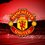# Poof of Dirichlet series of Riemann zeta function

Proof of: $\zeta \left( s \right) =\frac { 1 }{ s-1 } \sum _{ k=1 }^{ \infty }{ \left( \frac { k }{ { \left( k+1 \right) }^{ s } } -\frac { k-s }{ { k }^{ s } } \right) }$

Now,

$\displaystyle \zeta \left( s \right) =\sum _{ k=1 }^{ \infty }{ \frac { 1 }{ { k }^{ s } } }$

$\displaystyle \zeta \left( s \right) = s \int _{ 1 }^{ \infty }{ \frac { \left\lfloor t \right\rfloor }{ { t }^{ s+1 } } dt }$

$\displaystyle \zeta \left( s \right) = s \int _{ 1 }^{ \infty }{ \frac { t-\left\{ t \right\} }{ { t }^{ s+1 } } dt }$

$\displaystyle \zeta \left( s \right) = s \sum _{ k=1 }^{ \infty }{ \left( \int _{ k }^{ k+1 }{ \frac { t-t+k }{ { t }^{ s+1 } } dt } \right) }$

$\displaystyle \zeta \left( s \right) =s\sum _{ k=1 }^{ \infty }{ \left( \int _{ k }^{ k+1 }{ \frac { k }{ { t }^{ s+1 } } dt } \right) }$

$\displaystyle \zeta \left( s \right) =-\frac { 1 }{ 1 } \sum _{ k=1 }^{ \infty }{ \left( \frac { k }{ { \left( k+1 \right) }^{ s } } -\frac { k }{ { \left( k \right) }^{ s } } \right) }$

$\displaystyle \zeta \left( s \right) =\frac { 1 }{ s-1 } \sum _{ k=1 }^{ \infty }{ \left( \frac { k }{ { \left( k+1 \right) }^{ s } } -\frac { k }{ { \left( k \right) }^{ s } } +\frac { s }{ { \left( k \right) }^{ s } } \right) }$

$\large \text{HENCE PROVED}$

##### ORIGINAL5 years, 6 months ago

This discussion board is a place to discuss our Daily Challenges and the math and science related to those challenges. Explanations are more than just a solution — they should explain the steps and thinking strategies that you used to obtain the solution. Comments should further the discussion of math and science.

When posting on Brilliant:

• Use the emojis to react to an explanation, whether you're congratulating a job well done , or just really confused .
• Ask specific questions about the challenge or the steps in somebody's explanation. Well-posed questions can add a lot to the discussion, but posting "I don't understand!" doesn't help anyone.
• Try to contribute something new to the discussion, whether it is an extension, generalization or other idea related to the challenge.

MarkdownAppears as
*italics* or _italics_ italics
**bold** or __bold__ bold
- bulleted- list
• bulleted
• list
1. numbered2. list
1. numbered
2. list
Note: you must add a full line of space before and after lists for them to show up correctly
paragraph 1paragraph 2

paragraph 1

paragraph 2

[example link](https://brilliant.org)example link
> This is a quote
This is a quote
    # I indented these lines
# 4 spaces, and now they show
# up as a code block.

print "hello world"
# I indented these lines
# 4 spaces, and now they show
# up as a code block.

print "hello world"
MathAppears as
Remember to wrap math in $$ ... $$ or $ ... $ to ensure proper formatting.
2 \times 3 $2 \times 3$
2^{34} $2^{34}$
a_{i-1} $a_{i-1}$
\frac{2}{3} $\frac{2}{3}$
\sqrt{2} $\sqrt{2}$
\sum_{i=1}^3 $\sum_{i=1}^3$
\sin \theta $\sin \theta$
\boxed{123} $\boxed{123}$

Sort by:

Nice.. If you elaborate more on how you get 2nd line from 1st line . it will help beginners.

I hope you will elaborate more

- 5 years, 6 months ago

I've proved it in the solution of this problem.

- 5 years, 6 months ago